# RD Sharma Solutions for Class 11 Chapter 29 - Limits Exercise 29.4

This exercise’s main insight is to evaluate the algebra of limits using rationalisation method. Students who wish to strengthen their concepts can access the RD Sharma Class 11 Maths Solutions. To know the right methodology to solve questions of this exercise, students can refer and download the RD Sharma Solutions for Class 11 Maths Chapter 29 Limits Exercise 29.4 pdf, from the below provided links. Moreover, students can start practising offline to achieve their goals.

## Download the pdf of RD Sharma Solutions for Class 11 Maths Exercise 29.4 Chapter 29 – Limits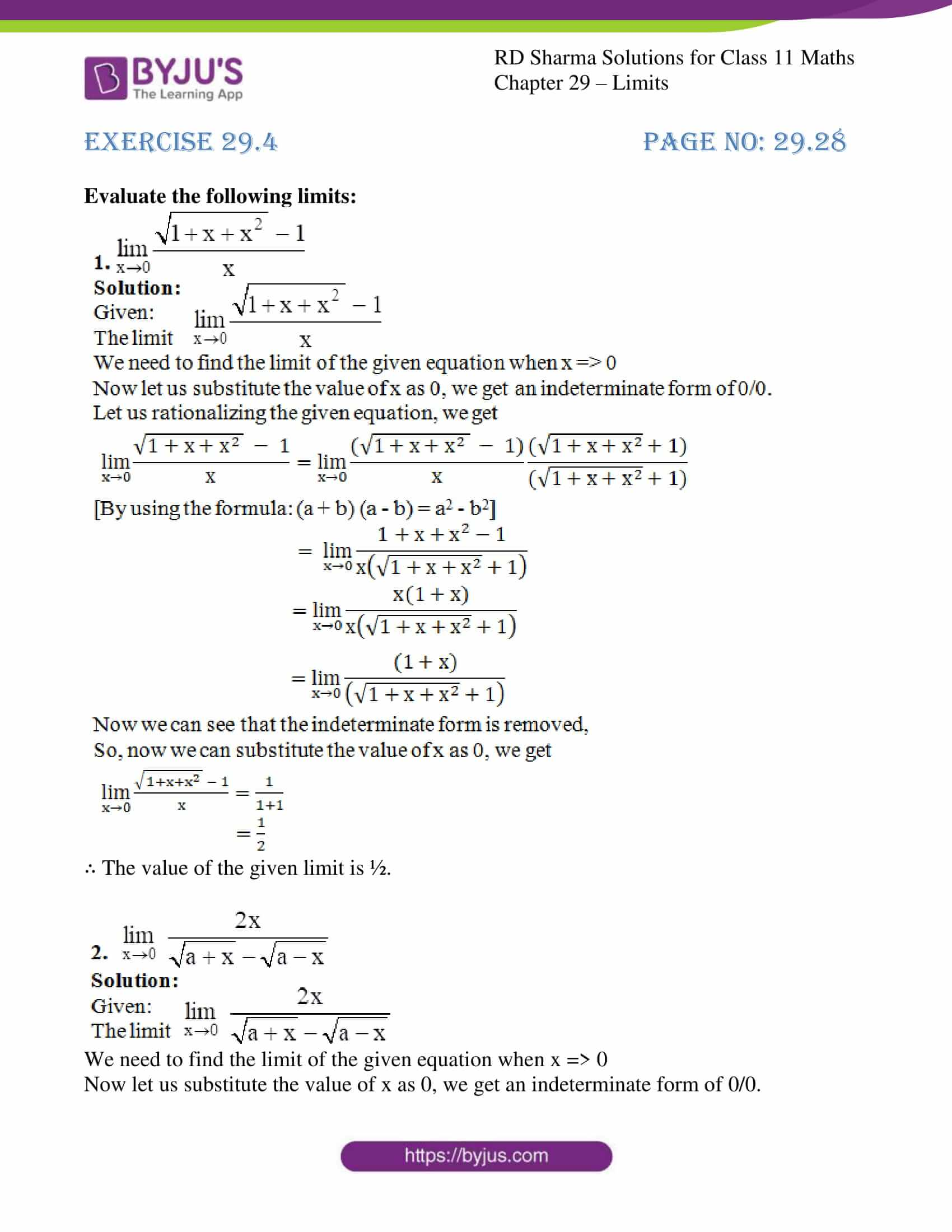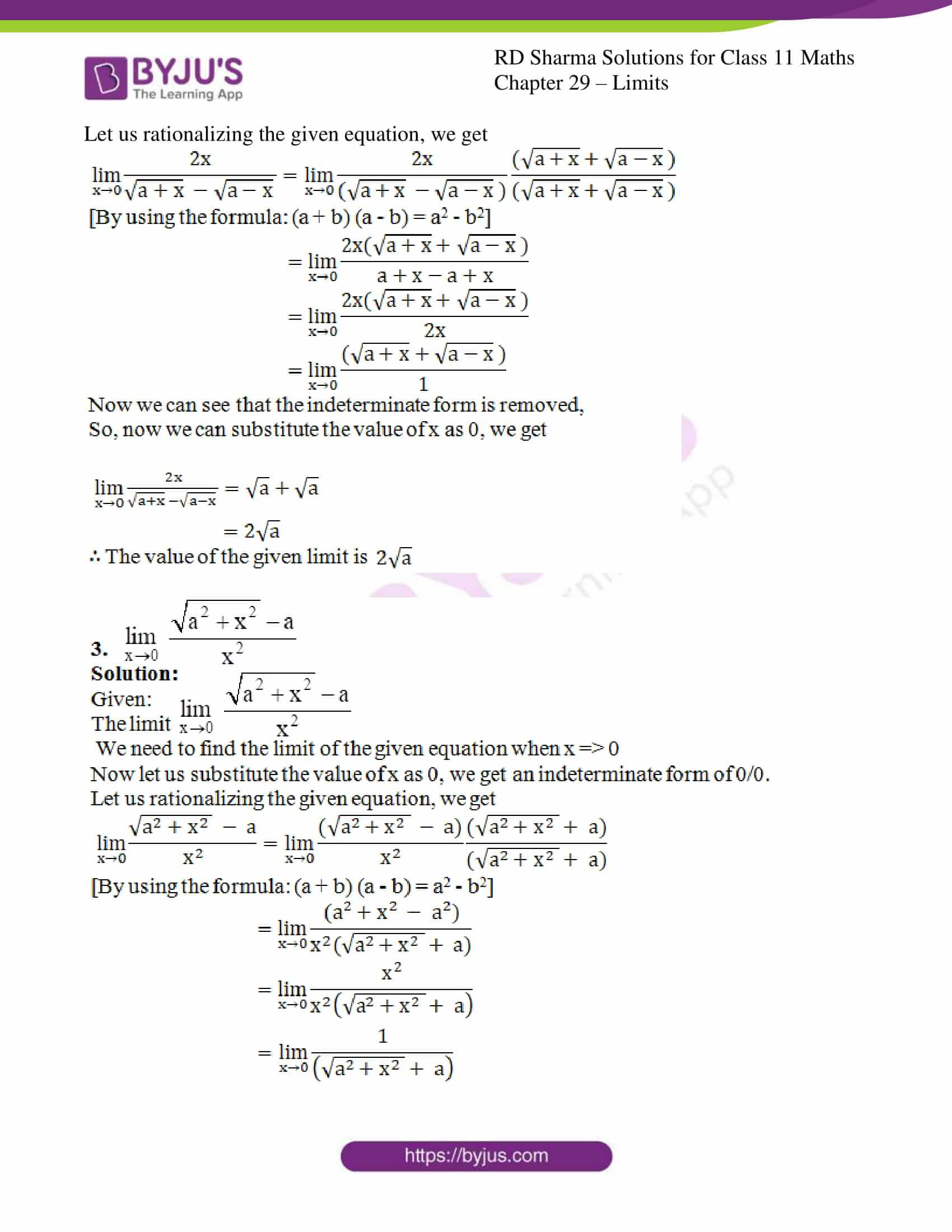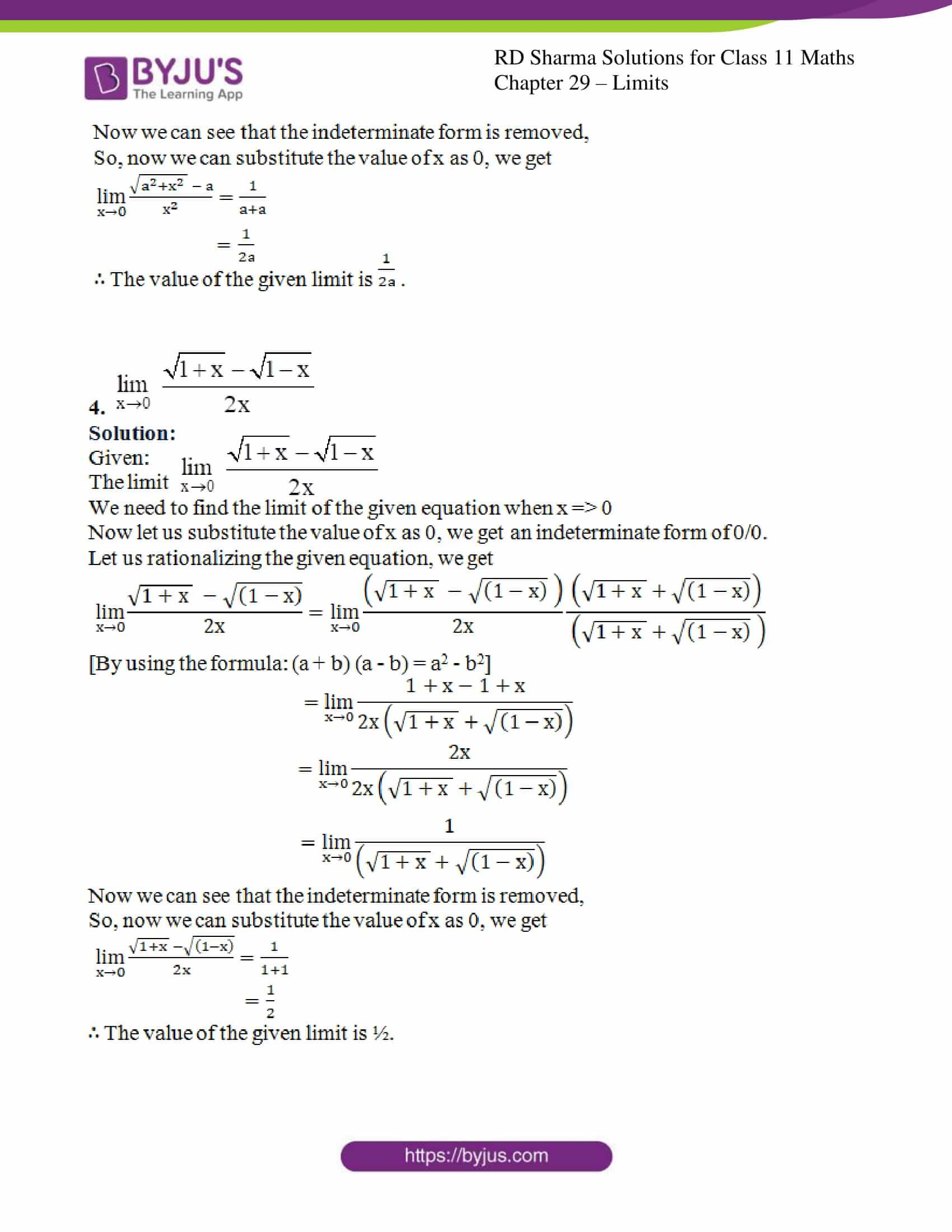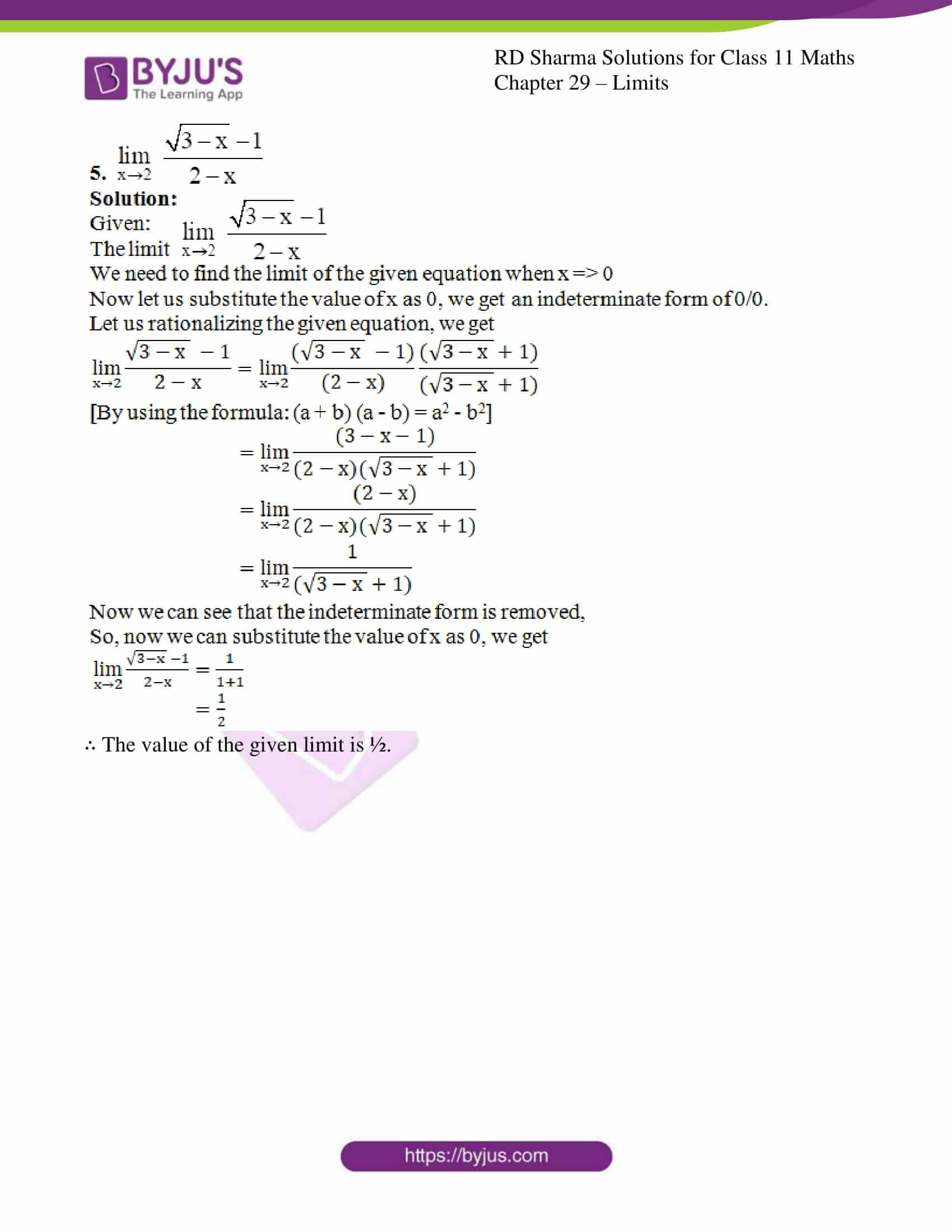### Access answers to RD Sharma Solutions for Class 11 Maths Exercise 29.4 Chapter 29 – Limits

#### EXERCISE 29.4 PAGE NO: 29.28

Evaluate the following limits: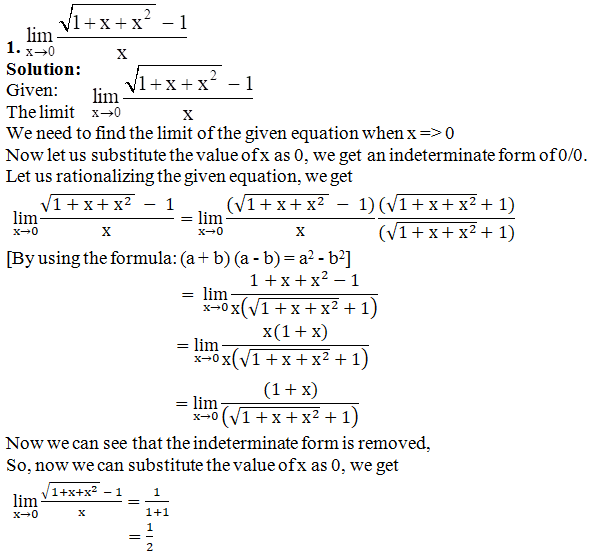∴ The value of the given limit is ½.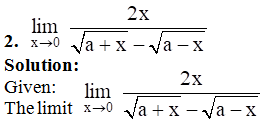We need to find the limit of the given equation when x => 0

Now let us substitute the value of x as 0, we get an indeterminate form of 0/0.

Let us rationalizing the given equation, we get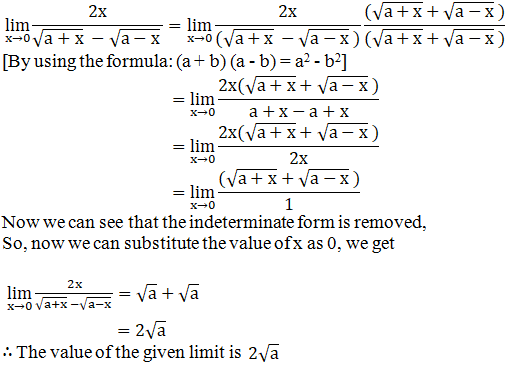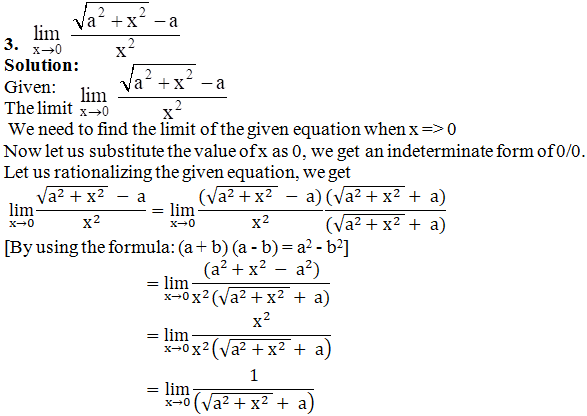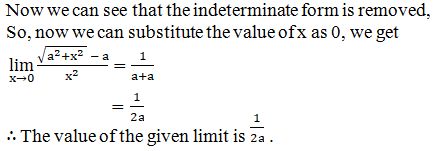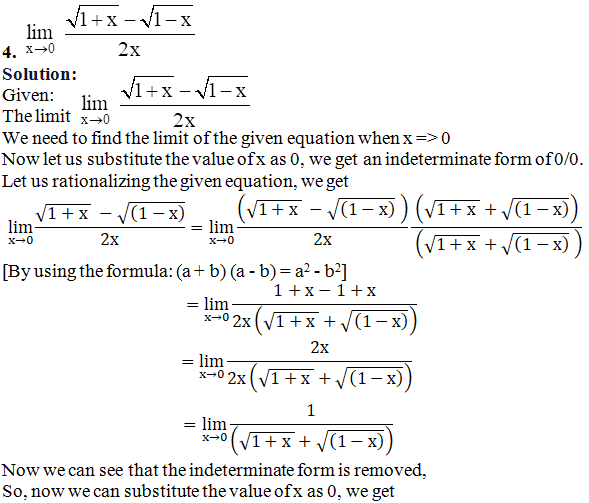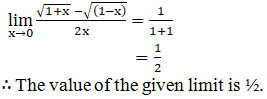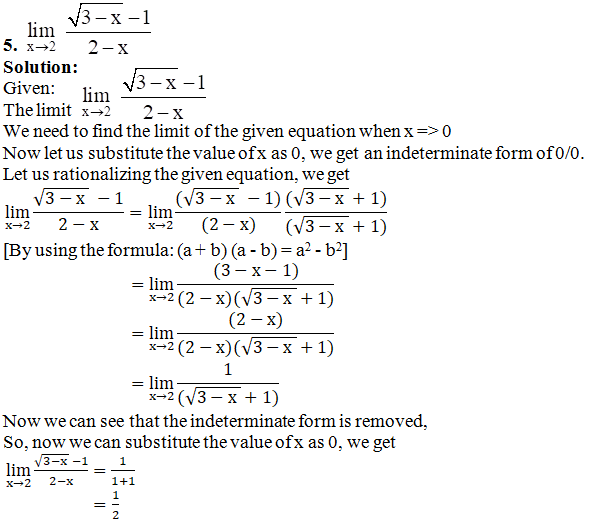∴ The value of the given limit is ½.